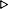ToC DocOverview CGDoc RelNotes Index PermutedIndex
Allegro CLANSI Common Lisp22 Printer22.4 Dictionary of Printer

 22.4.26 *print-pretty* Variable

Value Type:
a generalized boolean.

Initial Value:
implementation-dependent.

Description:
Controls whether the Lisp printer calls the pretty printer.

If it is false, the pretty printer is not used and a minimum of whitespace1 is output when printing an expression.

If it is true, the pretty printer is used, and the Lisp printer will endeavor to insert extra whitespace1 where appropriate to make expressions more readable.

*print-pretty* has an effect even when the value of *print-escape* is false.

Examples:
``` (setq *print-pretty* 'nil)NIL
(progn (write '(let ((a 1) (b 2) (c 3)) (+ a b c))) nil)(LET ((A 1) (B 2) (C 3)) (+ A B C))NIL
(let ((*print-pretty* t))
(progn (write '(let ((a 1) (b 2) (c 3)) (+ a b c))) nil))(LET ((A 1)(B 2)(C 3))(+ A B C))NIL
;; Note that the first two expressions printed by this next form
;; differ from the second two only in whether escape characters are printed.
;; In all four cases, extra whitespace is inserted by the pretty printer.
(flet ((test (x)
(let ((*print-pretty* t))
(print x)
(format t "~%~S " x)
(terpri) (princ x) (princ " ")
(format t "~%~A " x))))
(test '#'(lambda () (list "a" #\b 'c #'d))))#'(LAMBDA ()(LIST "a" #\b 'C #'D))#'(LAMBDA ()(LIST "a" #\b 'C #'D))#'(LAMBDA ()(LIST a b 'C #'D))#'(LAMBDA ()(LIST a b 'C #'D))NIL
```